# Multi Layer Network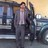Visiting Faculty Membor at International Islamic University à International Islamic University
23 Apr 2015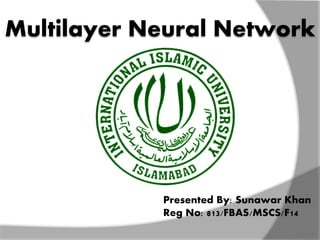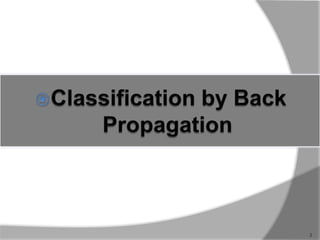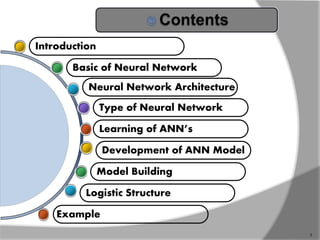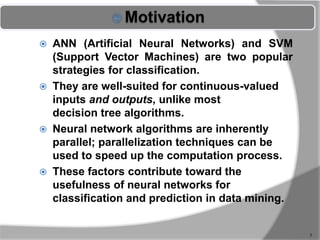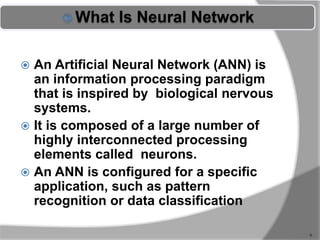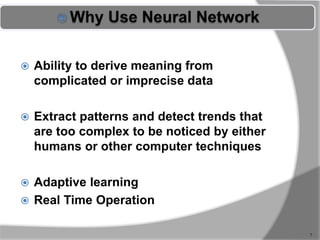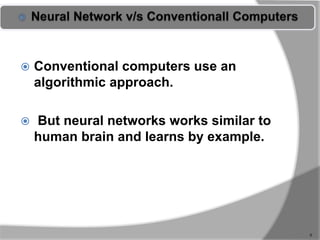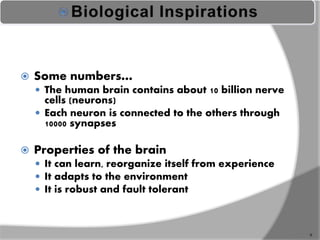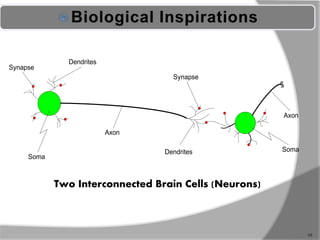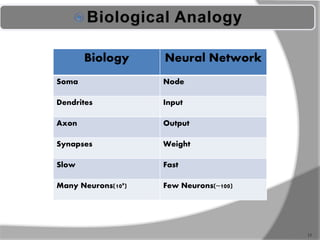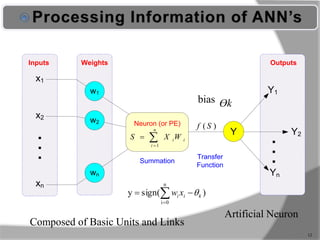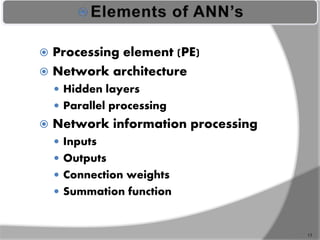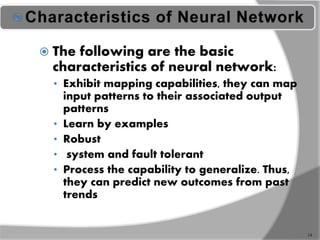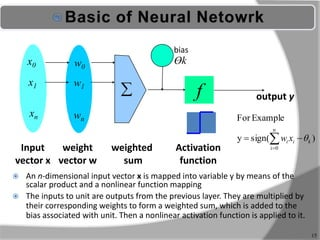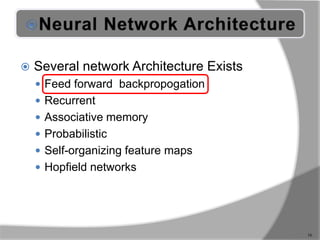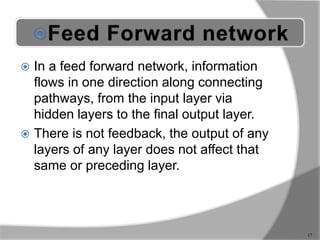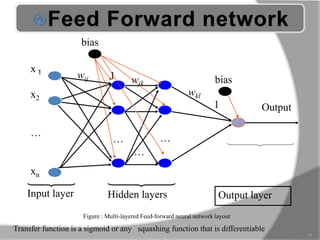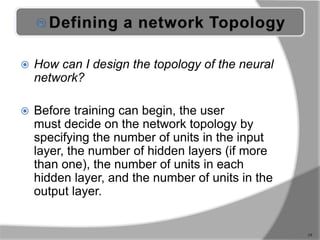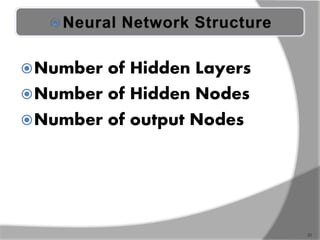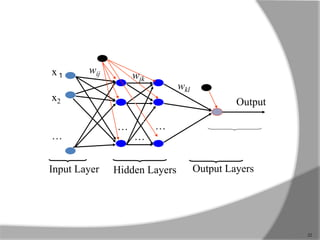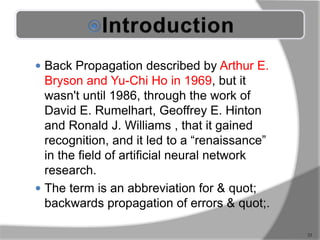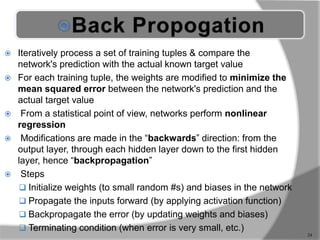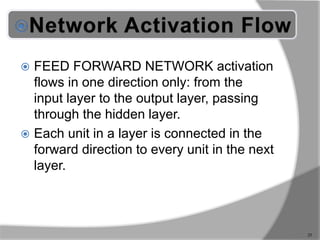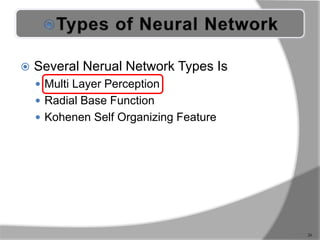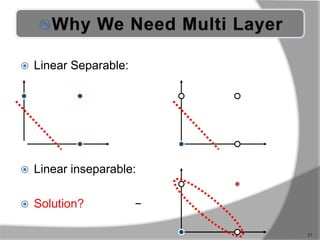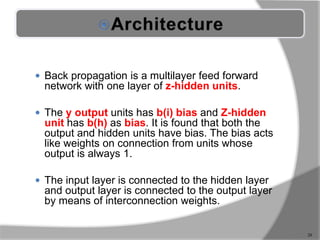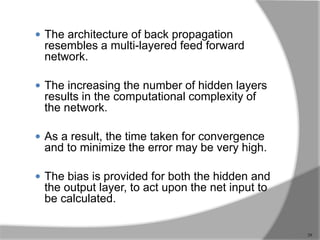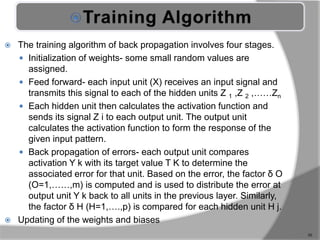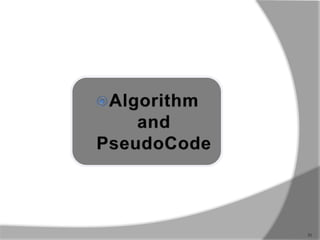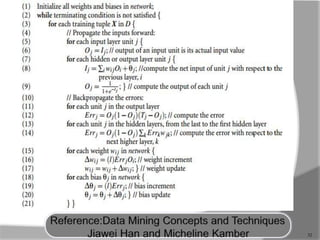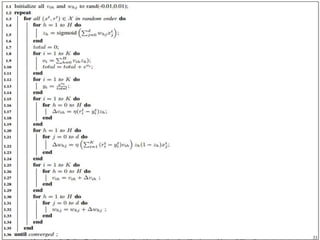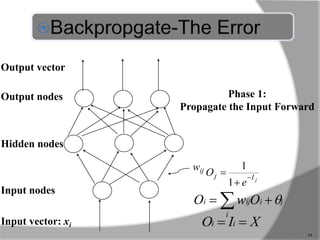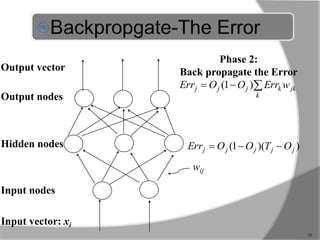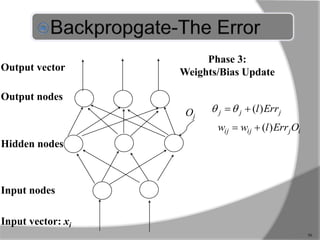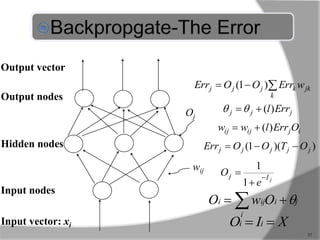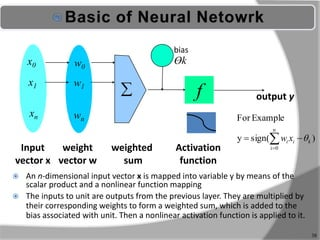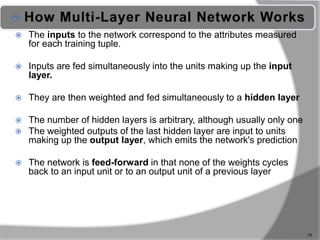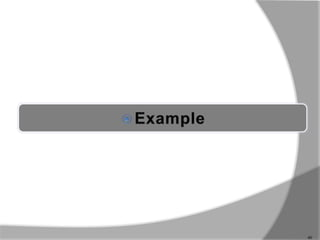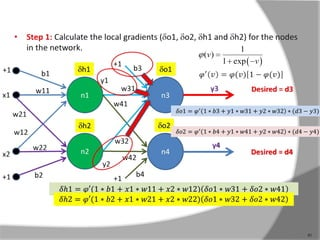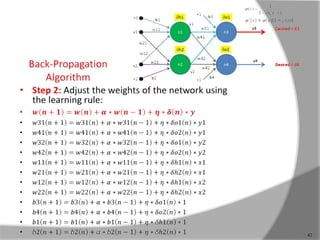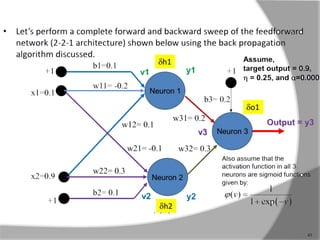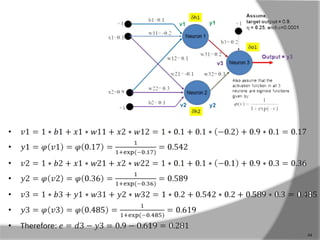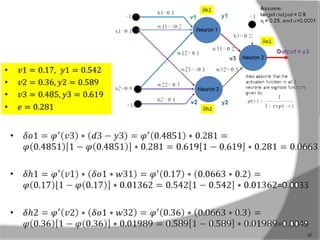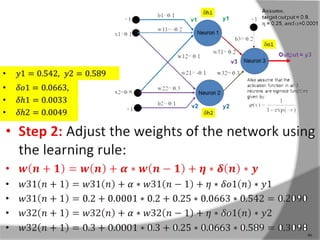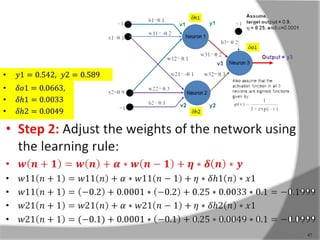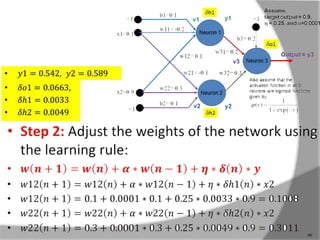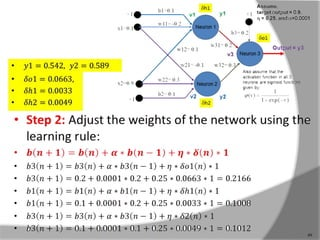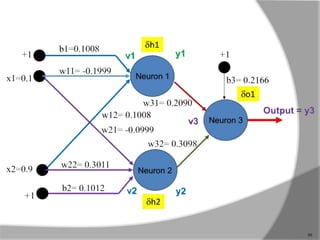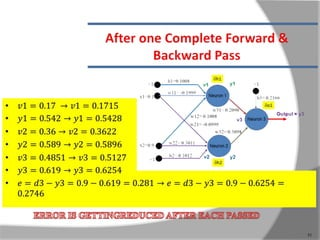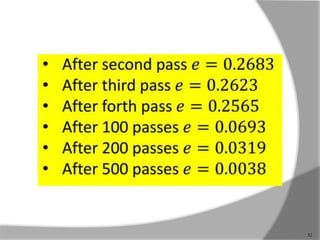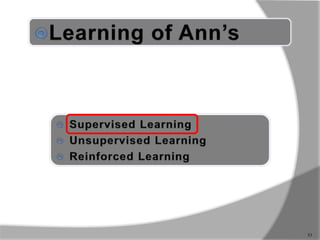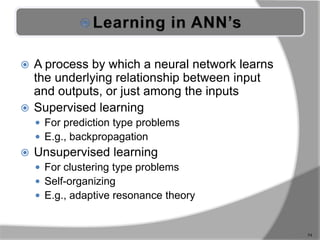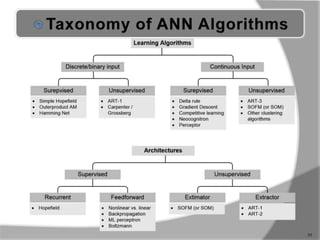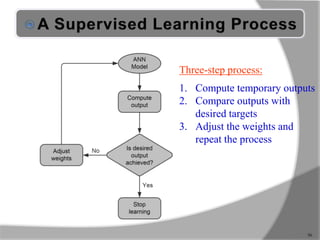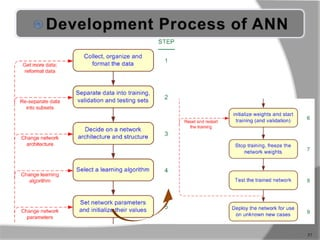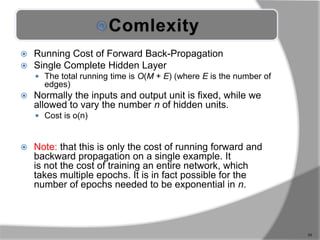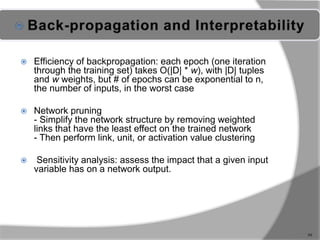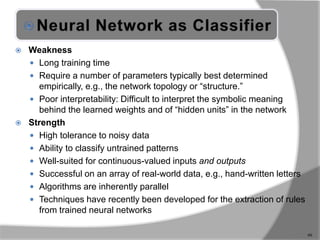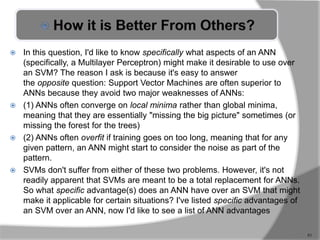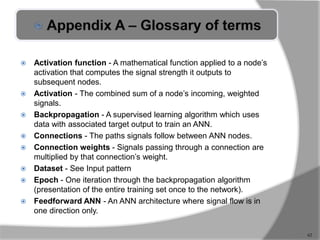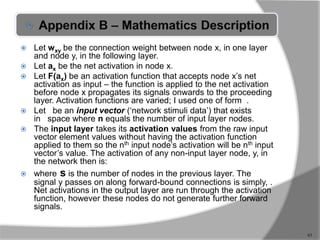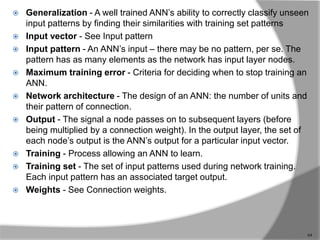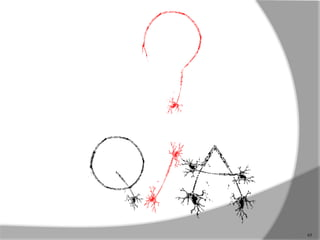1 sur 65

### Multi Layer Network

• 1. Multilayer Neural Network Presented By: Sunawar Khan Reg No: 813/FBAS/MSCS/F14
• 2. 2
• 3. Learning of ANN’s Type of Neural Network Neural Network Architecture Basic of Neural Network Introduction Logistic Structure Model Building Development of ANN Model Example 3
• 4. 4
• 5.  ANN (Artificial Neural Networks) and SVM (Support Vector Machines) are two popular strategies for classification.  They are well-suited for continuous-valued inputs and outputs, unlike most decision tree algorithms.  Neural network algorithms are inherently parallel; parallelization techniques can be used to speed up the computation process.  These factors contribute toward the usefulness of neural networks for classification and prediction in data mining. 5
• 6.  An Artificial Neural Network (ANN) is an information processing paradigm that is inspired by biological nervous systems.  It is composed of a large number of highly interconnected processing elements called neurons.  An ANN is configured for a specific application, such as pattern recognition or data classification 6
• 7.  Ability to derive meaning from complicated or imprecise data  Extract patterns and detect trends that are too complex to be noticed by either humans or other computer techniques  Adaptive learning  Real Time Operation 7
• 8.  Conventional computers use an algorithmic approach.  But neural networks works similar to human brain and learns by example. 8
• 9. 9  Some numbers…  The human brain contains about 10 billion nerve cells (neurons)  Each neuron is connected to the others through 10000 synapses  Properties of the brain  It can learn, reorganize itself from experience  It adapts to the environment  It is robust and fault tolerant
• 11. 11 Biology Neural Network Soma Node Dendrites Input Axon Output Synapses Weight Slow Fast Many Neurons(109) Few Neurons(~100)
• 12. 12 w1 w2 wn x1 x2 xn . . . Y Y1 Yn Y2 Inputs Weights Outputs . . . Neuron (or PE)   n i iiWXS 1 )( Sf Summation Transfer Function bias Artificial Neuron )sign(y n 0i kii xw   Ɵk Composed of Basic Units and Links
• 13. 13  Processing element (PE)  Network architecture  Hidden layers  Parallel processing  Network information processing  Inputs  Outputs  Connection weights  Summation function
• 14. 14  The following are the basic characteristics of neural network: • Exhibit mapping capabilities, they can map input patterns to their associated output patterns • Learn by examples • Robust • system and fault tolerant • Process the capability to generalize. Thus, they can predict new outcomes from past trends
• 15. 15  An n-dimensional input vector x is mapped into variable y by means of the scalar product and a nonlinear function mapping  The inputs to unit are outputs from the previous layer. They are multiplied by their corresponding weights to form a weighted sum, which is added to the bias associated with unit. Then a nonlinear activation function is applied to it. Ɵk f weighted sum Input vector x output y Activation function weight vector w  w0 w1 wn x0 x1 xn )sign(y ExampleFor n 0i kii xw   bias
• 16.  Several network Architecture Exists  Feed forward backpropogation  Recurrent  Associative memory  Probabilistic  Self-organizing feature maps  Hopfield networks 16
• 17.  In a feed forward network, information flows in one direction along connecting pathways, from the input layer via hidden layers to the final output layer.  There is not feedback, the output of any layers of any layer does not affect that same or preceding layer. 17
• 18. Output x１ x2 … xn bias biaswij Input layer Hidden layers Output layer … … … wjk wkl Transfer function is a sigmoid or any squashing function that is differentiable 1 1 Figure : Multi-layered Feed-forward neural network layout 18
• 19.  How can I design the topology of the neural network?  Before training can begin, the user must decide on the network topology by specifying the number of units in the input layer, the number of hidden layers (if more than one), the number of units in each hidden layer, and the number of units in the output layer. 19
• 20.  First decide the network topology : # of units in the input layer, # of hidden layer(if>1), # of units in each hidden layer, and # of units in the output layer.  Normalizing the input values for each attribute measured in the training tuples to [0.0-1.0]  One input unit per domain value, each initialized to 0  Output, if for classification and more than two classes, one output unit per class is used.  Once a network has been trained and its accuracy is unacceptable, repeat the training process with a different network topology or a different set of initial weights 20
• 21. Number of Hidden Layers Number of Hidden Nodes Number of output Nodes 21
• 22. 22 Output x１ x2 … wij Input Layer Hidden Layers … … … wjk wkl Output Layers
• 23.  Back Propagation described by Arthur E. Bryson and Yu-Chi Ho in 1969, but it wasn't until 1986, through the work of David E. Rumelhart, Geoffrey E. Hinton and Ronald J. Williams , that it gained recognition, and it led to a “renaissance” in the field of artificial neural network research.  The term is an abbreviation for & quot; backwards propagation of errors & quot;. 23
• 24.  Iteratively process a set of training tuples & compare the network's prediction with the actual known target value  For each training tuple, the weights are modified to minimize the mean squared error between the network's prediction and the actual target value  From a statistical point of view, networks perform nonlinear regression  Modifications are made in the “backwards” direction: from the output layer, through each hidden layer down to the first hidden layer, hence “backpropagation”  Steps  Initialize weights (to small random #s) and biases in the network  Propagate the inputs forward (by applying activation function)  Backpropagate the error (by updating weights and biases)  Terminating condition (when error is very small, etc.) 24
• 25.  FEED FORWARD NETWORK activation flows in one direction only: from the input layer to the output layer, passing through the hidden layer.  Each unit in a layer is connected in the forward direction to every unit in the next layer. 25
• 26.  Several Nerual Network Types Is  Multi Layer Perception  Radial Base Function  Kohenen Self Organizing Feature 26
• 27.  Linear Separable:  Linear inseparable:  Solution? yx  yx  yx  27
• 28.  Back propagation is a multilayer feed forward network with one layer of z-hidden units.  The y output units has b(i) bias and Z-hidden unit has b(h) as bias. It is found that both the output and hidden units have bias. The bias acts like weights on connection from units whose output is always 1.  The input layer is connected to the hidden layer and output layer is connected to the output layer by means of interconnection weights. 28
• 29.  The architecture of back propagation resembles a multi-layered feed forward network.  The increasing the number of hidden layers results in the computational complexity of the network.  As a result, the time taken for convergence and to minimize the error may be very high.  The bias is provided for both the hidden and the output layer, to act upon the net input to be calculated. 29
• 30.  The training algorithm of back propagation involves four stages.  Initialization of weights- some small random values are assigned.  Feed forward- each input unit (X) receives an input signal and transmits this signal to each of the hidden units Z 1 ,Z 2 ,……Zn  Each hidden unit then calculates the activation function and sends its signal Z i to each output unit. The output unit calculates the activation function to form the response of the given input pattern.  Back propagation of errors- each output unit compares activation Y k with its target value T K to determine the associated error for that unit. Based on the error, the factor δ O (O=1,……,m) is computed and is used to distribute the error at output unit Y k back to all units in the previous layer. Similarly, the factor δ H (H=1,….,p) is compared for each hidden unit H j.  Updating of the weights and biases 30
• 31. 31
• 32. 32
• 34. Output nodes Input nodes Hidden nodes Output vector Input vector: xi wij jIj e O    1 1 34 XIO ii  ji i iji OwO   Phase 1: Propagate the Input Forward
• 35. Output nodes Input nodes Hidden nodes Output vector Input vector: xi wij ))(1( jjjjj OTOOErr  jk k kjjj wErrOOErr  )1( 35 Phase 2: Back propagate the Error
• 36. Output nodes Input nodes Hidden nodes Output vector Input vector: xi Oj 36 ijijij OErrlww )( jjj Errl)( Phase 3: Weights/Bias Update
• 37. Output nodes Input nodes Hidden nodes Output vector Input vector: xi wij jIj e O    1 1 ))(1( jjjjj OTOOErr  jk k kjjj wErrOOErr  )1( ijijij OErrlww )( jjj Errl)( 37 XIO ii  ji i iji OwO   Oj
• 38. 38  An n-dimensional input vector x is mapped into variable y by means of the scalar product and a nonlinear function mapping  The inputs to unit are outputs from the previous layer. They are multiplied by their corresponding weights to form a weighted sum, which is added to the bias associated with unit. Then a nonlinear activation function is applied to it. Ɵk f weighted sum Input vector x output y Activation function weight vector w  w0 w1 wn x0 x1 xn )sign(y ExampleFor n 0i kii xw   bias
• 39.  The inputs to the network correspond to the attributes measured for each training tuple.  Inputs are fed simultaneously into the units making up the input layer.  They are then weighted and fed simultaneously to a hidden layer  The number of hidden layers is arbitrary, although usually only one  The weighted outputs of the last hidden layer are input to units making up the output layer, which emits the network's prediction  The network is feed-forward in that none of the weights cycles back to an input unit or to an output unit of a previous layer 39
• 40. 40
• 41. 41
• 42. 42
• 43. 43
• 44. 44
• 45. 45
• 46. 46
• 47. 47
• 48. 48
• 49. 49
• 50. 50
• 51. 51
• 52. 52
• 53. 53
• 54.  A process by which a neural network learns the underlying relationship between input and outputs, or just among the inputs  Supervised learning  For prediction type problems  E.g., backpropagation  Unsupervised learning  For clustering type problems  Self-organizing  E.g., adaptive resonance theory 54
• 55. 55
• 56. Three-step process: 1. Compute temporary outputs 2. Compare outputs with desired targets 3. Adjust the weights and repeat the process 56
• 57. 57
• 58.  Running Cost of Forward Back-Propagation  Single Complete Hidden Layer  The total running time is O(M + E) (where E is the number of edges)  Normally the inputs and output unit is fixed, while we allowed to vary the number n of hidden units.  Cost is o(n)  Note: that this is only the cost of running forward and backward propagation on a single example. It is not the cost of training an entire network, which takes multiple epochs. It is in fact possible for the number of epochs needed to be exponential in n. 58
• 59.  Efficiency of backpropagation: each epoch (one iteration through the training set) takes O(|D| * w), with |D| tuples and w weights, but # of epochs can be exponential to n, the number of inputs, in the worst case  Network pruning - Simplify the network structure by removing weighted links that have the least effect on the trained network - Then perform link, unit, or activation value clustering  Sensitivity analysis: assess the impact that a given input variable has on a network output. 59
• 60. 60  Weakness  Long training time  Require a number of parameters typically best determined empirically, e.g., the network topology or “structure.”  Poor interpretability: Difficult to interpret the symbolic meaning behind the learned weights and of “hidden units” in the network  Strength  High tolerance to noisy data  Ability to classify untrained patterns  Well-suited for continuous-valued inputs and outputs  Successful on an array of real-world data, e.g., hand-written letters  Algorithms are inherently parallel  Techniques have recently been developed for the extraction of rules from trained neural networks
• 61.  In this question, I'd like to know specifically what aspects of an ANN (specifically, a Multilayer Perceptron) might make it desirable to use over an SVM? The reason I ask is because it's easy to answer the opposite question: Support Vector Machines are often superior to ANNs because they avoid two major weaknesses of ANNs:  (1) ANNs often converge on local minima rather than global minima, meaning that they are essentially "missing the big picture" sometimes (or missing the forest for the trees)  (2) ANNs often overfit if training goes on too long, meaning that for any given pattern, an ANN might start to consider the noise as part of the pattern.  SVMs don't suffer from either of these two problems. However, it's not readily apparent that SVMs are meant to be a total replacement for ANNs. So what specific advantage(s) does an ANN have over an SVM that might make it applicable for certain situations? I've listed specific advantages of an SVM over an ANN, now I'd like to see a list of ANN advantages 61
• 62.  Activation function - A mathematical function applied to a node’s activation that computes the signal strength it outputs to subsequent nodes.  Activation - The combined sum of a node’s incoming, weighted signals.  Backpropagation - A supervised learning algorithm which uses data with associated target output to train an ANN.  Connections - The paths signals follow between ANN nodes.  Connection weights - Signals passing through a connection are multiplied by that connection’s weight.  Dataset - See Input pattern  Epoch - One iteration through the backpropagation algorithm (presentation of the entire training set once to the network).  Feedforward ANN - An ANN architecture where signal flow is in one direction only. 62
• 63.  Let wxy be the connection weight between node x, in one layer and node y, in the following layer.  Let ax be the net activation in node x.  Let F(ax) be an activation function that accepts node x’s net activation as input – the function is applied to the net activation before node x propagates its signals onwards to the proceeding layer. Activation functions are varied; I used one of form .  Let be an input vector (‘network stimuli data’) that exists in space where n equals the number of input layer nodes.  The input layer takes its activation values from the raw input vector element values without having the activation function applied to them so the nth input node’s activation will be nth input vector’s value. The activation of any non-input layer node, y, in the network then is:  where s is the number of nodes in the previous layer. The signal y passes on along forward-bound connections is simply, . Net activations in the output layer are run through the activation function, however these nodes do not generate further forward signals. 63
• 64.  Generalization - A well trained ANN’s ability to correctly classify unseen input patterns by finding their similarities with training set patterns  Input vector - See Input pattern  Input pattern - An ANN’s input – there may be no pattern, per se. The pattern has as many elements as the network has input layer nodes.  Maximum training error - Criteria for deciding when to stop training an ANN.  Network architecture - The design of an ANN: the number of units and their pattern of connection.  Output - The signal a node passes on to subsequent layers (before being multiplied by a connection weight). In the output layer, the set of each node’s output is the ANN’s output for a particular input vector.  Training - Process allowing an ANN to learn.  Training set - The set of input patterns used during network training. Each input pattern has an associated target output.  Weights - See Connection weights. 64
• 65. 65